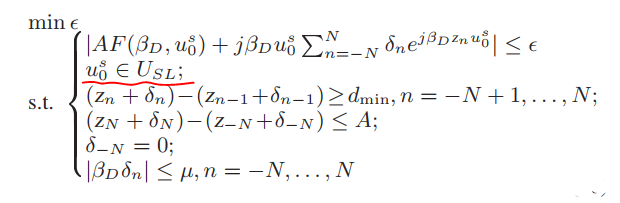# How to specify range of a parameter , thanksHi experts,

    I am new to convex optimization using CVX and I have to solve the above convex problem, the only variable is the 2N+1 element vector delta n. Other function like AF or parameters like betaD, Zn,dmin,N are all given. But in the problem, I need to specify the range of a parameter u0s (which is red highlighted),  it means for all u0s in this range, the top expression should be valid.  I am wondering how should I specify this range in CVX, should I specify u0s as a cvx variable? Thanks

This is “your” problem (presumably taken from a book or paper), not mine. I don’t understand what the notation means, and in particular, what is meant by the “constraint” (is it?) you highlighted. You say that \delta is the only variable, but clearly \epsilon is a variable.

Your first step is to completely understand the optimization problem you wish to solve. That is not a CVX matter.

@Mark_L_Stone Thanks for your prompt reply. Sorry for the inconvenience. The highlighted constraint means parameter u0s has the range of USL. USL is a given range. For any u0s in the range of USL, the top expression should be valid.
I think ϵ can be removed, the optimization goal is equivalent to directly minimize the absolute value of that top expression. So δ can be the only variable Many thanks

What you have provided is much too vague for me to know what it is supposed to mean. For instance, I have no idea what form AF(beta,ustar) takes, so no idea what any of this really means. i don’t even know what you mean by parameter - is that an input value or an optimization 9i.e., decision) variable?

If U were an optimization variable (scalar, vector, or matrix), bound constraints could be specified as lower_bound <= U <= upper_bound

@Mark_L_Stone

Thanks a lot. AF(beta, u0s) is a given function. AF as a functional of u0s. u0s is not an optimization variable, it is an input given value, but can be any value within the range defined by USL (for example 0 <u0s <80).  Question here is how to specify the range of this input value in CVX? Thanks


Best,

If it is an input value, you don’t tell CVX anything about it, other than it having whatever numerical value it has in MATLAB.

Your description continues to be too vague and generic. Unless you provided a detailed and complete exposition of the relevant functions, it will likely continue that way.

CVX is a finite, not infinite, dimensional solver. If you have a constraint which must hold for an infinite range of possible parameter values, that is not a finite dimensional constraint. Either the parameter must be made into an optimization variable, or a (possibly large) finite number of constraints must be specified in CVX, corresponding to different parameter values - whether that adequately captures the original problem is another matter.

Feel free to make U a CvX variable. But if that makes the first constraint non-convex or not compatible with CVX’s rules, that is tough luck, and it may be that CVX is not an appropriate tool for the problem.